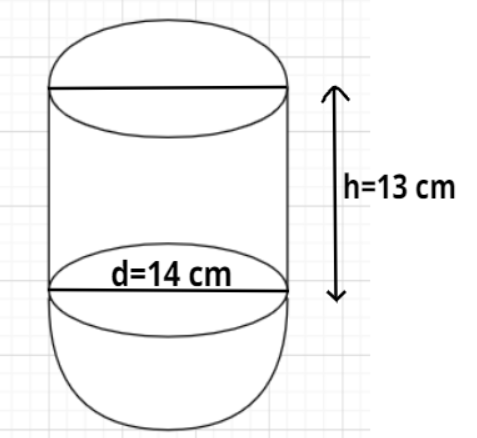Courses
Courses for Kids
Free study material
Free LIVE classes
MoreLIVE
Join Vedantu’s FREE Mastercalss

# A vessel in the form of a hollow hemisphere mounted by a hollow cylinder. The diameter of the hemisphere is 14 cm and the height of the cylindrical part is 13 cm. Find the inner surface area of the vessel.Verified
362.1k+ views
Hint- Here, we will be using the formulas for the curved surface area of the cylinder and hemisphere with the help of which we can determine the total inner surface area of the vessel.

Given, diameter of the hemispherical part of the vessel $d = 14{\text{ cm}}$
Height of the cylindrical part of the vessel $h = 13{\text{ cm}}$
Since, radius$= \dfrac{{{\text{diameter}}}}{2}$
So, radius of the hemispherical part of the vessel $r = \dfrac{d}{2} = \dfrac{{14}}{2} = 7{\text{ cm}}$
Since, the cylinder is mounted on the hemisphere so the diameters of both will be equal which means the radius of the cylindrical part will be equal to the radius of hemispherical part of the vessel.
i.e., Radius of the cylindrical part of the vessel $r = 7{\text{ cm}}$
Clearly, the inner surface of the vessel consists of the curved surface of the hollow hemispherical part and the curved surface of the cylindrical part of the vessel.
As we know that the curved surface area of the hemisphere having radius $r$ is $2\pi {r^2}$ and the curved surface area of the cylinder having radius $r$ and height $h$ is $2\pi rh$.
So, Inner surface area of the vessel$=$Curved surface area of the hemispherical part$+$Curved surface area of the cylindrical part
$\Rightarrow {\text{Inner surface area of the vessel}} = 2\pi {r^2} + 2\pi rh = 2\pi r\left( {r + h} \right) = 2 \times \dfrac{{22}}{7} \times 7 \times \left( {7 + 13} \right) = 44 \times 20 = 880{\text{ c}}{{\text{m}}^2}$
Hence, the inner surface area of the vessel is 880 cm2.

Note- In these types of problems, the figure is analysed carefully so that it follows the problem statement. In this particular problem, the cylinder is mounted on the hemispherical part of vessel so the base of the cylinder will merge with the open surface of the hemispherical part of the vessel and hence the radius of the cylindrical part and that of the hemispherical part will be equal to each other.
Last updated date: 23rd Sep 2023
Total views: 362.1k
Views today: 4.62k